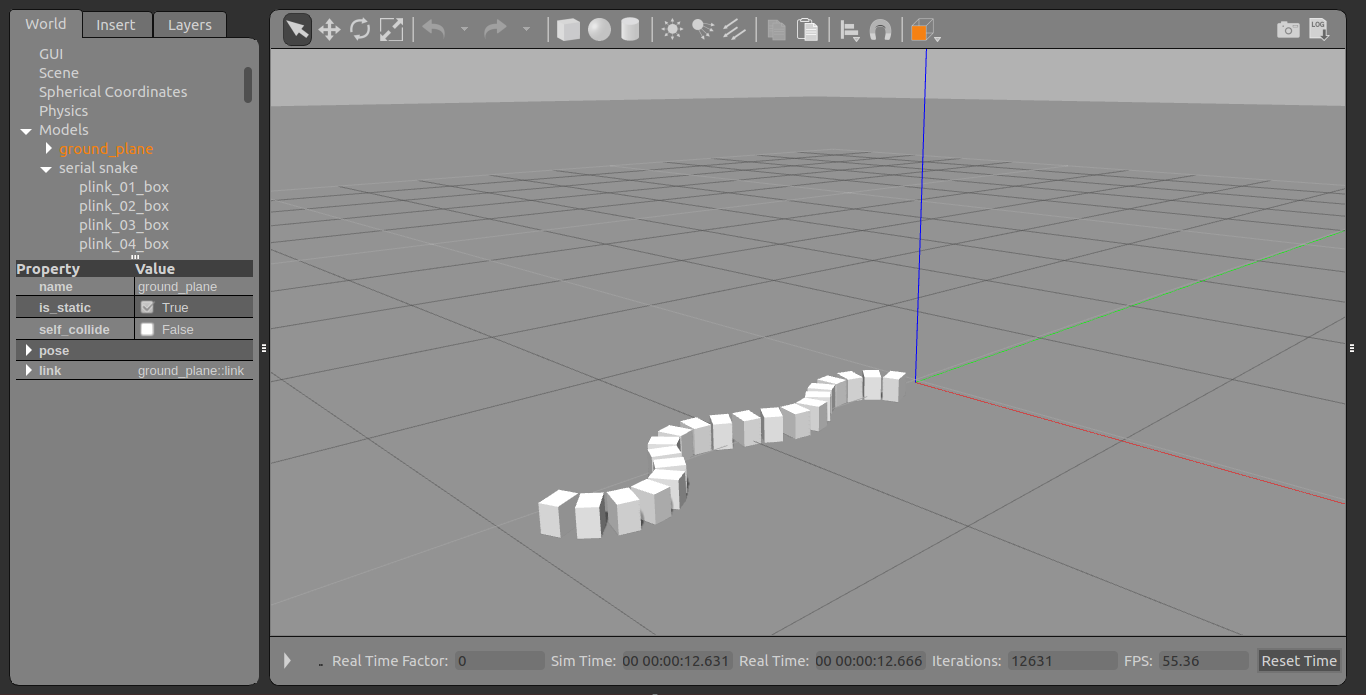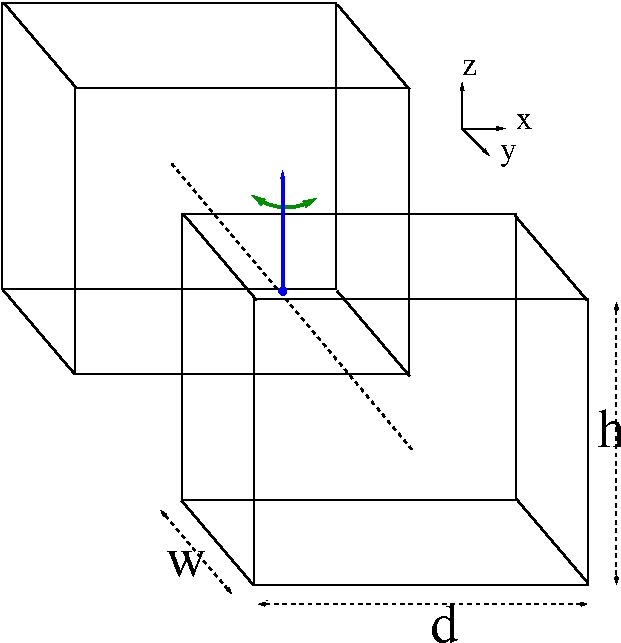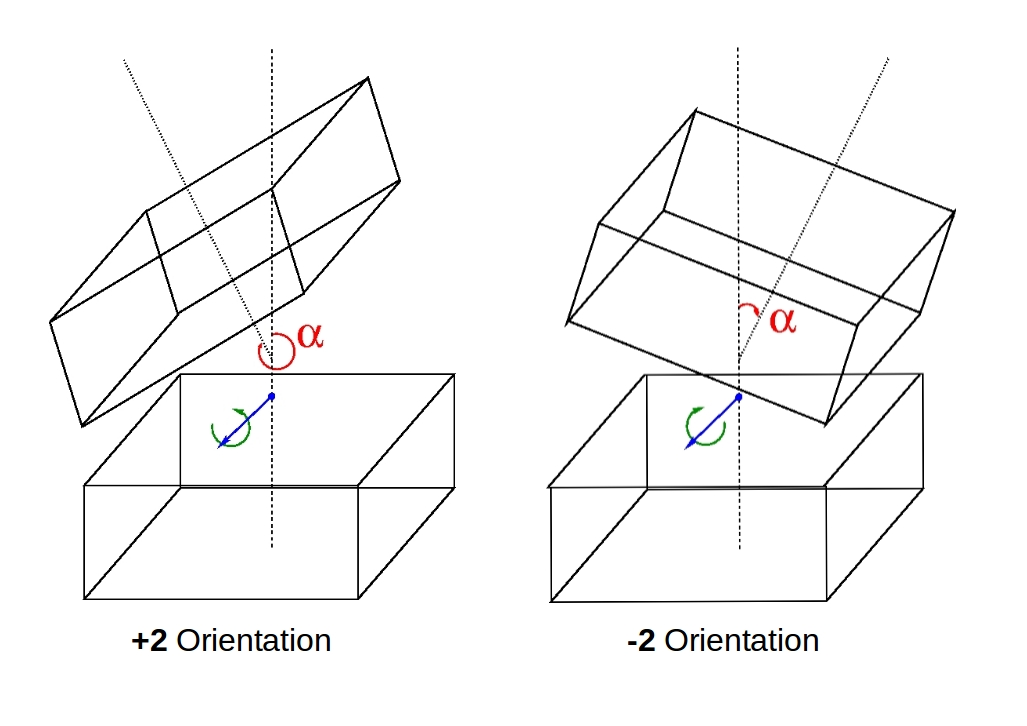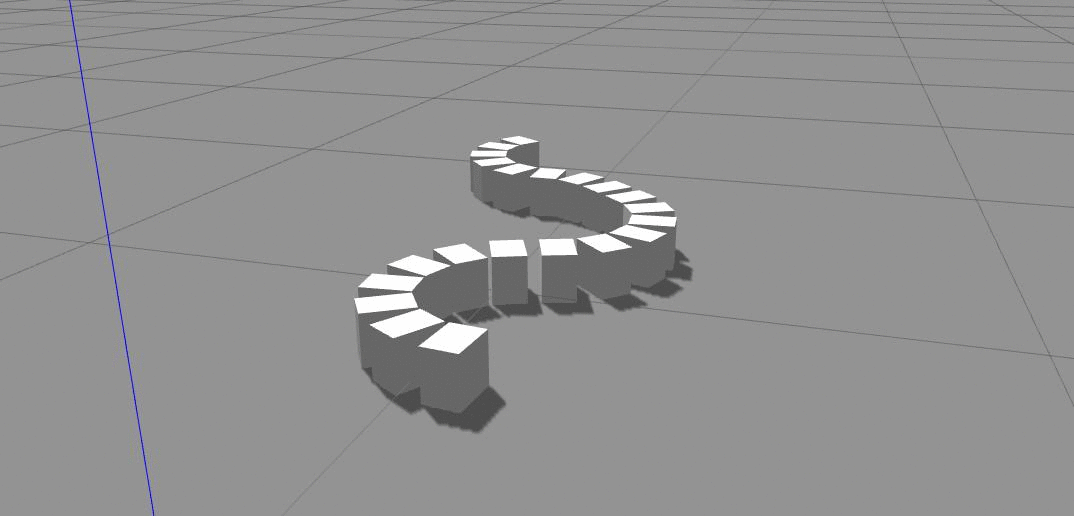I’ve always been fascinated by robots and well snakes. When it came to selecting a theme for my undergraduate research project I naturally ended up selecting an option which fits well with both. What started out as a research project sort of ended up being one my hobbies, so I’ll time and again update on my snake robot progress through my blog.

Now first to start off, essentially what I have been trying to do is to replicate serpentine motion in robots using simulations. Note that none of the methods I have developed have been experimentally tested. Maybe I’ll do it someday but there is still a long way to go before that… u’ll see why.

#### Prerequisites (Skip if you’ve used Gazebo or know some robotics)

Before I go further into what I’ve done, here are some things you’ll need to know.

• All my simulations were carried out using Gazebo, an open source software platform widely used in robotic simulations. Simulations are a cool way of testing out new ideas with minimal cost, and its way more cooler since it involves robots. If you’ve never used Gazebo then click here. The official website has all the instruction on how to install Gazebo and tutorials on how to get started.
• Also you’ll need to have some idea about robotics in general. Theory of Applied Robotics: Kinematics, Dynamics and Control is a great book you could use as a reference, but for now just knowing how to use Gazebo would be sufficient to understand what I’ve done.

#### The serial snake robot model

I made some assumptions when designing my initial snake robots purely to reduce the complexity of the motion. I assume that biological snakes move in a planar surface (2D) replicating a sinusoidal pattern, that scales don’t contribute to overall motion (which is false :)) and that a snakes body could be approximated by a linear combination of cuboids which are linked by revolute joints which rotate about the Z axis as shown below.<!-- ------------ serial link 17 ---------- -->
<pose>0 -1.60 0.05 0 0 0</pose> <!-- changes with -0.10 for each  y coord -->
<inertial>
<inertia>
<ixx>0.00018625</ixx> <!-- using tensor matrix -->
<iyy>0.00025</iyy>
<izz>0.00018625</izz>
<ixy>0</ixy>
<ixz>0</ixz>
<iyz>0</iyz>
</inertia>
<mass>0.15</mass>
</inertial>
<collision name="collision">
<geometry>
<box>
<size>0.1 0.07 0.1</size>
</box>
</geometry>
</collision>
<visual name="visual">
<geometry>
<box>
<size>0.1 0.07 0.1</size>
</box>
</geometry>
</visual>


I defined four main operations for the twist motion namely +2 and -2 which represent the maximum/minimum twist to the left and right respectively and +1,-1 which represents the twist to the left & right for half the maximum angle. For each cuboid I’ve defined a hard limit of maximum and minimum twist angles in the model description itself as shown below.Operation Twist Direction Angle($\alpha$)
+2 Left 335$^{\circ}$
+1 Left 342.5$^{\circ}$
-1 Right 12.5$^{\circ}$
-2 Right 25$^{\circ}$
<!-- code for joint between link 5 and 6 -->
<pose>0 -0.0525 0 0 0 0</pose> <!-- position relative to head -->
<axis>
<xyz>0 0 1</xyz> <!-- rotating axis is Z -->
<limit>
<lower>-0.436332</lower> <!-- upper limit for alpha in radians -->
<upper>0.436332</upper> <!-- lower limit for alpha in radians -->
</limit>
</axis>
</joint>


The code snippets below show the implementation for these twist like motions for each joint within the main plugin for the model.

void movementPlugin::moveJointLeft(int _jointPos) // +2 orientation
{
math::Angle currentAngle = this->joints[_jointPos]->GetAngle(0);
if(currentAngle <maxLimit ){
joints[_jointPos]->SetForce(0,10);//left
}
}

void movementPlugin::moveJointRight(int _jointPos) // -2 orientation
{
math::Angle currentAngle = this->joints[_jointPos]->GetAngle(0);
if(currentAngle >minLimit ){
joints[_jointPos]->SetForce(0,-10);//right
}
}

void movementPlugin::shrinkJoint(int _jointPos) // -1 & +1 orientation
{
math::Angle currentAngle = this->joints[_jointPos]->GetAngle(0);
math::Angle upper = maxLimit/2;
math::Angle lower = minLimit/2;
if(currentAngle < lower ){
joints[_jointPos]->SetForce(0,-10);
}else if(currentAngle >upper){
joints[_jointPos]->SetForce(0,10);
}
}


Instead of defining a sinusoidal movement pattern for the overall robot, I replicated that movement by creating 8 states or orientations for the complete snake robot, where those states would be iteratively executed until we need the robot to stop. Now note that there is no feedback loop here, and neither dose the robot know where its going. The idea I wanted to test out here was the possibility of creating complex motion patterns (Lateral Undulation in this case) without the need for equations of motion or any sort of computation.

As per the code below for ease of execution I broke down the 20 joints into 4 main segments namely head(M1-M5), body1(M6-M10), body2(M11-M15), tail(M16-M21) where the operations of either moveJointRight(), moveJointLeft() or shrinkJoint() would be executed for a complete segment at a given iteration of the loop.

// for all these functions k represents the index to start
// and limit the number of joints to execute the movement function
void movementPlugin::left(int k,int limit)
{
for(int i=0;i<limit;i++){
//int loc=lamdaLimit[k]-i; //  B->F
int loc=lamdaLimit[k]+i; //    F->B
moveJointLeft(loc);
}
}

void movementPlugin::right(int k,int limit)
{
for(int i=0;i<limit;i++){
//int loc=lamdaLimit[k]-i; //  B->F
int loc=lamdaLimit[k]+i; //    F->B
moveJointRight(loc);
}
}

void movementPlugin::shrink(int k,int limit)
{
for(int i=0;i<limit;i++){
//int loc=lamdaLimit[k]-i; //  B->F
int loc=lamdaLimit[k]+i; //    F->B
shrinkJoint(loc);
}
}


The Table below represents the states and the code for the counter method I used to transition from one state to another. For every 150 iterations the sequence jumps one step forward(1->2 ect). Of all the 8 states, the first 4 are the initial configurations. These configurations (Config 1-4) were used to set initial positions of the links such that a stationary sine wave was shown when considering the overall snake robot shape. Afterwards the configurations from 5-8 were iteratively looped with each update cycle in the plugin.

Configurations Head (M1-M5) Body1 (M6-M10) Body2 (M11-M15) Tail (M16-M21)
Configuration 1       +2
Configuration 2     +2 -1
Configuration 3   +2 -1 -2
Configuration 4 +2 -1 -2 +1
Configuration 5 -1 -2 +1 +2
Configuration 6 -2 +1 +2 -1
Configuration 7 +1 +2 -1 -2
Configuration 8 +2 -1 -2 +1
// The OnUpdate() function runs continuously
void movementPlugin::OnUpdate()
{
//------[ B1-> B2 -> B3 -> B4 ]---------------------------
//-------initial config (1-4)-----------------------------
if(initialConfigCount<150){
left(k0,5);
initialConfigCount++;
}else if(initialConfigCount<300){
shrink(k0,5);
left(k1,5);
initialConfigCount++;
}else if(initialConfigCount<450){
right(k0,5);
shrink(k1,5);
left(k2,5);
initialConfigCount++;
}else if(initialConfigCount<600){
shrink(k0,5);
right(k1,5);
shrink(k2,5);
left(k3,5);
initialConfigCount++;
}else{
//-------continues config (5-8)-------------------------
if(sequenceCount==0){
left(k0,5);
shrink(k1,5);
right(k2,5);
shrink(k3,5);
count++;
}else if(sequenceCount==1){
shrink(k0,5);
left(k1,5);
shrink(k2,5);
right(k3,5);
count++;
}else if(sequenceCount==2){
right(k0,5);
shrink(k1,5);
left(k2,5);
shrink(k3,5);
count++;
}else if(sequenceCount==3){
shrink(k0,5);
right(k1,5);
shrink(k2,5);
left(k3,5);
count++;
}
}
//----------seconds counter-----------------------
if(count>150){
count=0;
sequenceCount=(sequenceCount+1)%4;
}
}


And thats it, The gif below shows the snake robot simulation as described by the code above. So basically it is in-fact possible to replicate complex biological motion from simple methods like this instead of relying purely on mathematical computations. However this is far from perfect. Right now this snake has no idea where its going, and neither is it capable of controlling its speed or optimizing its movement based on environmental constraints. So in future I’d probably try to implement this method on other designs and try to increase the efficiency of the movement.If you’d like to work on this code or need this for some other project feel free to get it from here and if you want more recent updates on my snake robots before it becomes a post follow the project on ResearchGate.

So until next time,